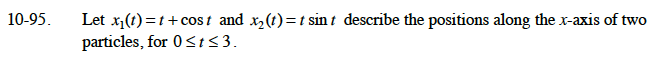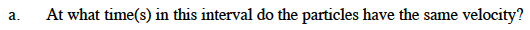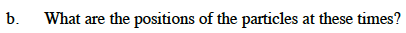### Home > CALC > Chapter Ch10 > Lesson 10.2.1 > Problem10-95

10-95.
1. Let x1 (t) = t + cos t and x2 (t) = t sin t describe the positions along the x-axis of two particles, for 0 ≤ t ≤ 3. Homework Help ✎

1. At what time(s) in this interval do the particles have the same velocity?

2. What are the positions of the particles at these times?Evaluate the original (position) equations for the value of t you obtained in part (a).